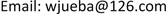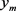﻿ 劳动力摩擦及其产业间配置与经济增长：一个基于两部门的研究 Labor Force Friction and Its Inter-Industry Allocation and Economic Growth: A Study Based on Two Sectors

Management Science and Engineering
Vol. 07  No. 04 ( 2018 ), Article ID: 28065 , 12 pages
10.12677/MSE.2018.74036

Labor Force Friction and Its Inter-Industry Allocation and Economic Growth: A Study Based on Two Sectors

Dongbei Bai, Jue Wang

School of Economics and Management, Northwest University, Xi’an ShaanxiReceived: Nov. 26th, 2018; accepted: Dec. 11th, 2018; published: Dec. 18th, 2018ABSTRACT

This paper constructs the model of labor allocation effect between two sectors, and uses the analysis methods of provincial static panel, provincial dynamic panel and provincial panel threshold effect to test the theoretical model. The results show that there is an inverted U-shaped relationship between the effect of labor force allocation between industries in the agricultural sector and non-agricultural sector on economic growth, and that the effect of labor force allocation between industries from the agricultural sector to the non-agricultural sector has a positive effect on economic growth in all the samples of central and western provinces. The inter-industry labor allocation effect of the transfer of labor force from agricultural sector to non-agricultural sector in eastern provinces inhibits economic growth. The negative impact of labor force frictions on economic growth is 37.5% of the total effect of inter-industry labor force allocation, and the negative impact of the eastern provinces is −32.8% larger than that of the whole sample and the central and western provinces. This shows that the eastern provinces have institutional obstacles to the inclusive employment of migrant workers. Obstacles have severely inhibited economic growth. Therefore, when the economy enters a new normal, reducing labor friction promotes the effect of inter-industry labor allocation, and provides a new way of thinking for the choice of economic growth path.

Keywords:Labor Mobility, Economic Growth, Inter-Industry Allocation, Labor FrictionCopyright © 2018 by authors and Hans Publishers Inc.1. 引言

2. 理论模型构建

$Y\left(t\right)=K{\left(t\right)}^{\alpha }{\left(A\left(t\right)\cdot L\left(t\right)\right)}^{\beta }$ (1)

$\frac{\Delta Y}{Y}=\alpha \frac{\Delta K}{K}+\beta \frac{\Delta L}{L}+\beta \frac{\Delta A}{A}$ (2)

$\frac{{W}_{m}}{{W}_{\alpha }}=\delta \left(1+\frac{1}{\psi }\frac{P}{1-P}\right)$ (3)

${y}_{\alpha }=\frac{{Y}_{\alpha }}{Y}$${y}_{m}=\frac{{Y}_{m}}{Y}$ ；其中：Y、 ${Y}_{\alpha }$${Y}_{m}$ 分别表示产出、农业部门产出和非农业部门产出； ${y}_{\alpha }$分别

$\frac{\stackrel{˙}{Y}}{Y}=\frac{{\stackrel{˙}{Y}}_{\alpha }}{{Y}_{\alpha }}\frac{{Y}_{\alpha }}{Y}+\frac{{\stackrel{˙}{Y}}_{m}}{{Y}_{m}}\frac{{Y}_{m}}{Y}={y}_{\alpha }\frac{{\stackrel{˙}{Y}}_{\alpha }}{{Y}_{\alpha }}+{y}_{m}\frac{{Y}_{m}}{Y}$ (4)

${y}_{j}\frac{{\stackrel{˙}{Y}}_{j}}{{Y}_{j}}={y}_{j}\frac{\partial {Y}_{j}}{\partial {K}_{j}}\frac{{K}_{j}}{{Y}_{j}}\frac{{\stackrel{˙}{K}}_{j}}{{K}_{j}}+{y}_{j}\frac{\partial {Y}_{j}}{\partial {L}_{j}}\frac{{L}_{j}}{{Y}_{j}}\frac{{\stackrel{˙}{L}}_{j}}{{L}_{j}}+{y}_{j}\frac{\partial {Y}_{j}}{\partial {A}_{j}}\frac{{A}_{j}}{{Y}_{j}}\frac{{\stackrel{˙}{A}}_{j}}{{A}_{j}}$ (5)

${y}_{\alpha }\frac{\partial {Y}_{\alpha }}{\partial {K}_{\alpha }}\frac{{K}_{\alpha }}{{Y}_{\alpha }}\frac{{\stackrel{˙}{K}}_{\alpha }}{{K}_{\alpha }}+{y}_{m}\frac{\partial {Y}_{m}}{\partial {K}_{m}}\frac{{K}_{m}}{{Y}_{m}}\frac{{\stackrel{˙}{K}}_{m}}{{K}_{m}}=r\frac{K}{Y}\frac{\stackrel{˙}{K}}{K}=\alpha \frac{\stackrel{˙}{K}}{K}$ (6)

$\frac{\stackrel{˙}{\stackrel{˜}{A}}}{\stackrel{˜}{A}}={y}_{\alpha }\frac{\partial {Y}_{\alpha }}{\partial {A}_{\alpha }}\frac{{\stackrel{˙}{A}}_{\alpha }}{{Y}_{\alpha }}+{y}_{m}\frac{\partial {Y}_{m}}{\partial {A}_{m}}\frac{{\stackrel{˙}{A}}_{m}}{{Y}_{m}}$ (7)

$\frac{\stackrel{˙}{Y}}{Y}=\alpha \frac{\stackrel{˙}{K}}{K}+{y}_{\alpha }\frac{\partial {Y}_{\alpha }}{\partial {L}_{\alpha }}\frac{{L}_{\alpha }}{{Y}_{\alpha }}\frac{{\stackrel{˙}{L}}_{\alpha }}{{L}_{\alpha }}+{y}_{m}\frac{\partial {Y}_{m}}{\partial {L}_{m}}\frac{{L}_{m}}{{Y}_{m}}\frac{{\stackrel{˙}{L}}_{m}}{{L}_{m}}+\frac{\stackrel{˙}{\stackrel{˜}{A}}}{\stackrel{˜}{A}}$ (8)

$\frac{{W}_{\alpha }{\stackrel{˙}{L}}_{\alpha }}{Y}+\frac{{W}_{m}{\stackrel{˙}{L}}_{m}}{Y}=\frac{{W}_{\alpha }L}{Y}\frac{\stackrel{˙}{L}}{L}+\frac{{W}_{m}L}{Y}\frac{{\stackrel{˙}{L}}_{m}}{L}-\frac{{W}_{\alpha }L}{Y}\frac{{\stackrel{˙}{L}}_{m}}{L}$ (9)

$\frac{{W}_{\alpha }{\stackrel{˙}{L}}_{\alpha }}{Y}+\frac{{W}_{m}{\stackrel{˙}{L}}_{m}}{Y}=\frac{{W}_{\alpha }L}{Y}\frac{\stackrel{˙}{L}}{L}+\frac{{W}_{\alpha }L}{Y}\left(\delta -1\right){l}_{m}\frac{{\stackrel{˙}{L}}_{m}}{{L}_{m}}+\frac{{W}_{\alpha }L}{Y}\delta \frac{1}{\psi }\frac{P}{1-P}{l}_{m}\frac{{\stackrel{˙}{L}}_{m}}{{L}_{m}}$ (10)

$\frac{{W}_{\alpha }L}{Y}\frac{\stackrel{˙}{L}}{L}=\beta \frac{\stackrel{˙}{L}}{L}+\frac{{W}_{\alpha }{L}_{m}-{W}_{m}{L}_{m}}{Y}\frac{\stackrel{˙}{L}}{L}$ (11)

$\frac{{W}_{\alpha }L}{Y}\frac{\stackrel{˙}{L}}{L}=\beta \frac{\stackrel{˙}{L}}{L}-\frac{{W}_{\alpha }L}{Y}\left(\delta -1\right){l}_{m}\frac{\stackrel{˙}{L}}{L}-\frac{{W}_{\alpha }L}{Y}\delta \frac{1}{\psi }\frac{P}{1-P}{l}_{m}\frac{\stackrel{˙}{L}}{L}$ (12)

$\frac{{W}_{\alpha }{\stackrel{˙}{L}}_{\alpha }}{Y}+\frac{{W}_{m}{\stackrel{˙}{L}}_{m}}{Y}=\beta \frac{\stackrel{˙}{L}}{L}+\frac{{W}_{\alpha }L}{Y}\left(\delta -1\right){l}_{m}\left(\frac{{\stackrel{˙}{L}}_{m}}{{L}_{m}}-\frac{\stackrel{˙}{L}}{L}\right)+\frac{{W}_{\alpha }L}{Y}\delta \frac{1}{\psi }\frac{P}{1-P}{l}_{m}\left(\frac{{\stackrel{˙}{L}}_{m}}{{L}_{m}}-\frac{\stackrel{˙}{L}}{L}\right)$

$\frac{{W}_{\alpha }{\stackrel{˙}{L}}_{\alpha }}{Y}+\frac{{W}_{m}{\stackrel{˙}{L}}_{m}}{Y}=\beta \frac{\stackrel{˙}{L}}{L}+\frac{{W}_{\alpha }L}{Y}\left(\delta -1\right){\stackrel{˙}{l}}_{m}+\frac{{W}_{\alpha }L}{Y}\delta \frac{1}{\psi }\frac{P}{1-P}{\stackrel{˙}{l}}_{m}$ (13)

$\frac{\stackrel{˙}{Y}}{Y}=\alpha \frac{\stackrel{˙}{K}}{K}+\beta \frac{\stackrel{˙}{L}}{L}+\frac{{W}_{\alpha }L}{Y}\left(\delta -1\right){\stackrel{˙}{l}}_{m}+\frac{{W}_{\alpha }L}{Y}\delta \frac{1}{\psi }\frac{P}{1-P}{\stackrel{˙}{l}}_{m}+\frac{\stackrel{˙}{\stackrel{˜}{A}}}{\stackrel{˜}{A}}$ (14)

3. 实证检验与结果分析

3.1. 指标说明与数据来源

1) 指标说明

ZGDP用以表示GDP增长速度。本文所计算的地区GDP增长率基于每个省、市、自治区的名义价格的生产总值存量。

ZK用以表示核心解释变量资本的增长率。资本存量测算采用单豪杰(2008)  的算法，用永续盘存法进行估算，估计公式为 ${K}_{t}={K}_{t-1}\left(1-\theta \right)+{I}_{t}$ ，式中 ${K}_{t}$ 为t时期的资本存量， $\theta$ 为折旧率(基期资本存量K1978使用单豪杰的估计结果，设定折旧率10.96%)； ${I}_{t}$ 为当年资本投资额。

ZL用以表示劳动增长率。通过各省市自治区的年末从业人员总数进行环比计算衡量从业劳动力增长率。农业部门向非农业部门转移的劳动力变量用LA来表示，通过非农业就业比重、劳动者报酬占比以及非农业劳动力增长率进行测算所得。存在劳动力摩擦的农业部门劳动力向非农业部门流动变量用MA来表示，通过劳动力流动P值与非农业劳动力增长率进行测算所得。

2) 数据来源

3.2. 计量模型构建

$ZGDP=\alpha +{\alpha }_{1}ZL+{\alpha }_{2}ZK+{\alpha }_{3}LA+{\alpha }_{4}MA+{\alpha }_{5}X+{\lambda }_{i}+{u}_{t}+{\epsilon }_{it}$ (15)

$ZGD{P}_{it}=\alpha +\rho ZGD{P}_{it-1}+{\beta }_{it}ZL+{\gamma }_{it}ZK+{\varphi }_{it}LA+{\phi }_{it}MA+{\nu }_{i}X+{u}_{i}+{\epsilon }_{it}$ (16)

$\begin{array}{c}ZGD{P}_{it}={u}_{i}+{\beta }_{1}Z{K}_{it}+{\beta }_{2}Z{L}_{it}+{\alpha }_{1}L{A}_{it}\cdot I\left(L{A}_{it}\le {\gamma }_{1}\right)+{\alpha }_{2}L{A}_{it}\cdot I\left(L{A}_{it}>{\gamma }_{1}\right)\\ \text{\hspace{0.17em}}\text{\hspace{0.17em}}+{\nu }_{1}M{A}_{it}\cdot I\left(L{A}_{it}\le {\gamma }_{1}\right)+{\nu }_{2}M{A}_{it}\cdot I\left(L{A}_{it}>{\gamma }_{1}\right)+\lambda {X}_{it}+{\epsilon }_{it}\end{array}$ (17)

3.3. 实证结果分析

1) 基准回归

2) 内生性与稳健性分析Table 3. Endogenous and robust discussions on measurement results

3) 地区差异性检验

4) 门槛效应检验

4. 结论

Labor Force Friction and Its Inter-Industry Allocation and Economic Growth: A Study Based on Two Sectors[J]. 管理科学与工程, 2018, 07(04): 297-308. https://doi.org/10.12677/MSE.2018.74036

1. 1. Denison, E. (1967) Why Growth Rates Differ: Postwar Experience in Nine Western Countries. Brooking Institution Press, Washington DC, 212-214.

2. 2. Denison, E. (1974) Accounting for United States Economic Growth 1929-1969. Brooking Institution Press, Washington DC, 287.

3. 3. Syrquin, M. (1984) Resource Reallocation and Productivity Growth in Hollis B, Economic Structure and Performance: Essays in Honour of Hollis B. Chenery. Academic Press, New York, 81.

4. 4. Dowrick, S. and Gemmel, N. (1991) Industrialization, Catching-Up, and Economic Growth: A Comparative Study across the World’s Capitalist Economies. The Economic Journal, 101, 263-275.
https://doi.org/10.2307/2233817

5. 5. Young, A. (2003) Gold into Base Metals: Productivity Growth in the People’s Republic of China during the Reform Period. Journal of Political Economy, 111, 1220-1261.
https://doi.org/10.1086/378532

6. 6. Aldrighi, D. and Colistete, R.P. (2013) Industrial Growth and Structural Change: Brazil in a Long-Run Perspective. Department of Economics_FEA/USP, Working Paper NO 3.

7. 7. Sepp, J. and Varblance, U. (2014) The Decomposition of Productivity Gap between Estonia and Korea. Ordnugspolitische Diskurse (OPO), Working Paper NO 3.

8. 8. 钱纳里, 赛尔昆. 发展的型式: 1950-1970 [M]. 北京 经济科学出版社, 1988: 34-78.

9. 9. 钱纳里, 赛尔昆. 工业化和经济增长的比较研究[M]. 上海: 三联书店, 1989: 31-89.

10. 10. 张保法. 经济增长中的结构效应[J]. 数量经济技术经济研究, 1997(11): 33-35.

11. 11. 潘文卿. 中国农业剩余劳动力转移效益测评[J]. 统计研究, 1999(4): 31-34.

12. 12. 蔡昉, 王德文. 中国经济增长可持续性与劳动贡献[J]. 经济研究, 1999(10): 62-68.

13. 13. 胡永泰. 中国全要素生产率: 来自农业部门劳动力再配置的首要作用[J]. 经济研究, 1998(3): 33-41.

14. 14. 徐现祥, 舒元. 劳动力结构效应的实证分析[J]. 上海经济研究, 2001(2): 9-14.

15. 15. 郝大明. 1978-2014年中国劳动力配置效应的分离与实证[J]. 经济研究, 2015, 7: 16-29.

16. 16. 辛超, 张平, 袁富华. 资本与劳动力配置结构效应——中国案例与国际比较[J]. 中国工业经济, 2015(2): 5-17.

17. 17. 单豪杰. 中国资本存量K的再估算: 1952-2006年[J]. 数量经济技术经济研究, 2008, 25(10): 17-31.

18. 18. Lin, J.Y. (2011) New Structural Economics: A Framework for Rethinking Development. The World Bank Research Observer, 26, 193-221.
https://doi.org/10.1093/wbro/lkr007

19. 19. Lin, J.Y. and Rosenblatt, D. (2012) Shifting Patterns of Economic Growth and rethinking Development. Journal of Economic Policy Reform, 15, 171-194.
https://doi.org/10.1080/17487870.2012.700565

20. 20. Massell, B.F. (1961) A Disaggregated View of Technical Change. Journal of Political Economy, 69, 547-557.
https://doi.org/10.1086/258575

21. 21. Denison, E. (1962) The Sources of Economic Growth in the United States and the Choices before US. Supplementary Paper.

22. 22. Denison, E. (1979) Accounting for Slower Economic Growth: The United States in the 1970s. The Brooking Institution Press, Washing DC, 65.